Lighthouse3d.comBugsLed Shader Tutorial# Led Shader Tutorial - Part II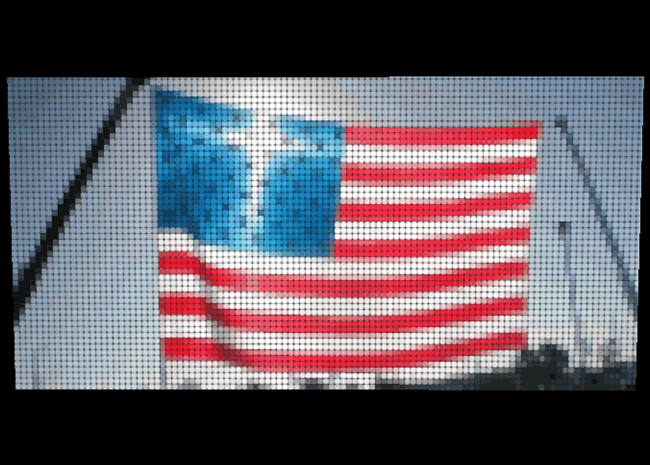### Better Pixelated Billboard

We saw in LED Shader Tutorial - Part I how we could take a texture and pixelate it, giving it that tiled look that you often get on large LED displays. We will now see how to make this look even better by making this pixelation circular and introducing some blending.

I think it is about time that I make the concrete case for this pixelation, as well as the change from a gridlike pixelation to a blended circular pixelation. Look that pictures below of actual LED displays. Notice that even the highest resolution graphic LED display has pixelation! And also notice that none of the pixelations are made up of perfect squares.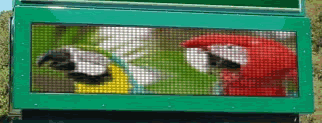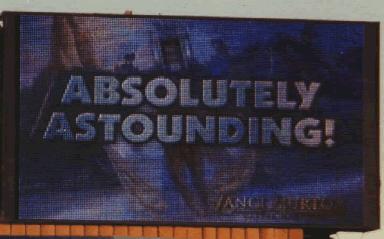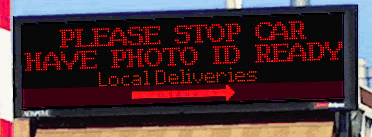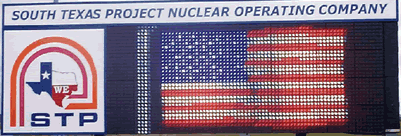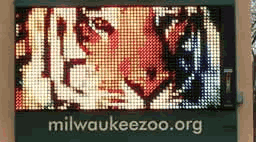So as you can see, this pixelation effect is very important. But our pixelation from LED Shader Tutorial - Part I was definitely not looking like this. Mainly, there were no black borders seperating the pixel regions, but also significant is the lack of rounded edges on our pixel regions.

New Uniforms used in the fragment program:
• float "pixelRadius" - radius of the circle defining our pixel regions. This should have a range [0.0 - 1.0] for reasons you will understand later. Beginning at 0.5 the circles will begin to hit the edge of their pixel regions and they'll look more like rounded squares. At 1.0 you will have a square.
• float "tolerance" - a value used to determine the gradient from pixel region color to the black used to seperate the regions. The higher you make this value the more blurry the edges of your circles will get.

I will now discuss two new devices we will need to implement these improvements. An equation for a circle and the GLSL function smoothstep.

Equation for a circle:
A circle is defined in cartesian coordinate as (x - h)2 + (y - k)2 = r2 where (h,k) is the center of the circle of radius r. As you may recall from LED Shader Tutorial - Part I we are mostly working in texture coordinates. The problem with this is that we have an elliptical coordinate system. Look at the figure below.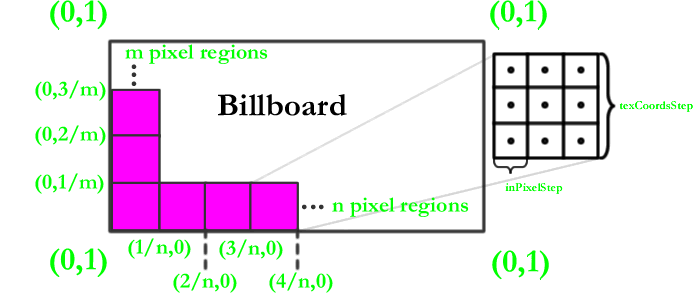You may remember me mentioning in the previous tutorial that texCoordsStep will likely be different in the x direction then the y direction. This is because the values m and n in the figure above will be different. Using texture coordinates directly as our coordinate system results in an elliptical system. This is a problem.

Imagine if we tried to use a base case + offset method similar to what we are doing for our sample points. So the center of our circle would be located at (inPixelStep.x + inPixelHalfStep.x, inPixelStep.y + inPixelHalfStep.y) and our radius wouldn't be constant! With out a constant radius we can't use the equation for a circle. So if we use this method we would have to use an equation for an ellipse: (x - h)2 / a2 + (y - k)2 / b2 = 1. This is much more computationally expensive and therefore undesirable. So we do something a little different. We add a variable:

• pixelRegionCoords - stores x and y coordinates in pixel region space.

This might seem confusing. Another coordinate space to think about. It turns out it is not that complicated. All we are doing is taking the fraction left over by the division we perform to get our pixel region bin.

vec2 pixelRegionCoords = fract(gl_TexCoord.st/texCoordsStep);

Now we can use our circle equation in conjunction with these pixelRegionCoords to make our pixel regions rounded! Note that this pixel region space will range from 0.0 to 1.0.

Smoothstep:
This is a built in GLSL function with the following specification: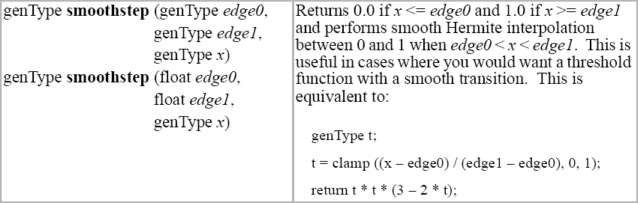So instead of assigning our pixel region color to all fragments in the circle and black to all fragments out of the circle, we use this function to get a blending of color from inside to outside.

So how do these two devices combine?
Look at the following code:

```	  ```
vec2 powers = pow(abs(pixelRegionCoords - 0.5),vec2(2.0));

```
```

First we compute (x - h)2 and (y - k)2 from our cirlce equation. Recall that (h,k) is the center of our circle. Since we are operating in pixel region coordinates, the center of our pixel region is (0.5,0.5). We take the abs(pixelRegionCoords - 0.5) simply due to a quark of the GLSL language. The pow(x,y) function is undefined for values of x < 0. We then compute r2 from our circle equation and then we are ready for smoothstep.

We smoothstep around our radiusSqrd value by using the tolerance variable described earlier. The higher this tolerance is, the larger a gradient we will get along the edge of our circle.

We use our gradient value to do a linear blend between our pixel region color and a very dark gray. This turns out quite nicely.

Conceptual summary of shader steps for each fragment (The current fragment being processed is referred to in the first person):

• compute base case sample locations in texture coordinates
• find out which pixel region I am in and apply that offset to the base case to get my sample locations
• use texture coordinates I have computed to get 9 color values from my pixel region
• store the average of those color values
• determine where I am in relation to the circle defining my pixel region
• use tolerance uniform and my position to get a gradient coeffecient
• I get assigned a blending of my pixel region color and dark gray based on the gradient coeffecient

Go to LED Shader Tutorial - Part III for a look at improving this technique!

### Advanced Topic Section This section is not needed to understand the tutorial.

Possible speed enhancements?
It should be noted that there are some speed enhancements that can be made to this shader. If the current fragment being processed will result in a gradient coeffecient = 0.0 (in other words, the fragment is completely outside of the circle and will have no pixel region color in it), we need not compute the pixel region color. This will rarely be the case, however, and it would make the code much less intuitive to read.

Another interesting enhancement would be to use some kind of texture as a means of determining the shape of each pixel region. Then you wouldn't be limited to the circle shape, you could easily do things with rounded rectangular regions, star formations (which are what some LEDs look like due to an arrangement of the LEDs into star-like clusters), etc.

The code (if you are going to use the code you should refer to the Source and Demo page):

```  ```
void main (void)
{
gl_TexCoord = gl_MultiTexCoord0;
gl_Position = ftransform();
}

/*************************************************************
*Email: jasejc 'at' aol.com
*CS594 University of Illinios at Chicago
*
*************************************************************/

#define KERNEL_SIZE 9

uniform int pixelSize; //size of bigger "pixel regions". These regions are forced to be square
uniform ivec2 billboardSize; //dimensions in pixels of billboardTexture
uniform sampler2D billboardTexture; //texure to be applied to billboard quad

// a tolerance used to determine the amount of blurring
// along the edge of the circle defining our "pixel region"
uniform float tolerance;

//the radius of the circle that will be our "pixel region", values > 0.5 hit the edge of the "pixel region"

vec2 texCoords[KERNEL_SIZE]; //stores texture lookup offsets from a base case

void main(void)
{
//will hold our averaged color from our sample points
vec4 avgColor;

//width of "pixel region" in texture coords
vec2 texCoordsStep = 1.0/(vec2(float(billboardSize.x),float(billboardSize.y))/float(pixelSize));

//x and y coordinates within "pixel region"
vec2 pixelRegionCoords = fract(gl_TexCoord.st/texCoordsStep);

//"pixel region" number counting away from base case
vec2 pixelBin = floor(gl_TexCoord.st/texCoordsStep);

//width of "pixel region" divided by 3 (for KERNEL_SIZE = 9, 3x3 square)
vec2 inPixelStep = texCoordsStep/3.0;
vec2 inPixelHalfStep = inPixelStep/2.0;

//use offset (pixelBin * texCoordsStep) from base case
// (the lower left corner of billboard) to compute texCoords
float offset = pixelBin * texCoordsStep;

texCoords = vec2(inPixelHalfStep.x, inPixelStep.y*2.0 + inPixelHalfStep.y) + offset;
texCoords = vec2(inPixelStep.x + inPixelHalfStep.x, inPixelStep.y*2.0 + inPixelHalfStep.y) + offset;
texCoords = vec2(inPixelStep.x*2.0 + inPixelHalfStep.x, inPixelStep.y*2.0 + inPixelHalfStep.y) + offset;
texCoords = vec2(inPixelHalfStep.x, inPixelStep.y + inPixelHalfStep.y) + offset;
texCoords = vec2(inPixelStep.x + inPixelHalfStep.x, inPixelStep.y + inPixelHalfStep.y) + offset;
texCoords = vec2(inPixelStep.x*2.0 + inPixelHalfStep.x, inPixelStep.y + inPixelHalfStep.y) + offset;
texCoords = vec2(inPixelHalfStep.x, inPixelHalfStep.y) + offset;
texCoords = vec2(inPixelStep.x + inPixelHalfStep.x, inPixelHalfStep.y) + offset;
texCoords = vec2(inPixelStep.x*2.0 + inPixelHalfStep.x, inPixelHalfStep.y) + offset;

//take average of 9 pixel samples
avgColor = texture2D(billboardTexture, texCoords) +
texture2D(billboardTexture, texCoords) +
texture2D(billboardTexture, texCoords) +
texture2D(billboardTexture, texCoords) +
texture2D(billboardTexture, texCoords) +
texture2D(billboardTexture, texCoords) +
texture2D(billboardTexture, texCoords) +
texture2D(billboardTexture, texCoords) +
texture2D(billboardTexture, texCoords);

avgColor /= float(KERNEL_SIZE);

//blend between fragments in the circle and out of the circle defining our "pixel region"
//Equation of a circle: (x - h)^2 + (y - k)^2 = r^2
vec2 powers = pow(abs(pixelRegionCoords - 0.5),vec2(2.0));